griddedInterpolant

Gridded data interpolation

Description

Use griddedInterpolant to perform interpolation on a 1-D, 2-D, 3-D, or N-D gridded data set. griddedInterpolant returns the interpolant F for the given data set. You can evaluate F at a set of query points, such as (xq,yq) in 2-D, to produce interpolated values vq = F(xq,yq).

Use scatteredInterpolant to perform interpolation with scattered data.

Creation

Description

F = griddedInterpolant creates an empty gridded data interpolant object.

example

F = griddedInterpolant(x,v) creates a 1-D interpolant from a vector of sample points x and corresponding values v.

example

F = griddedInterpolant(X1,X2,...,Xn,V) creates a 2-D, 3-D, or N-D interpolant using a full grid of sample points passed as a set of n-dimensional arrays X1,X2,...,Xn. The V array contains the sample values associated with the point locations in X1,X2,...,Xn. Each of the arrays X1,X2,...,Xn must be the same size as V.

example

F = griddedInterpolant(V) uses the default grid to create the interpolant. When you use this syntax, griddedInterpolant defines the grid as a set of points whose spacing is 1 and range is [1, size(V,i)] in the ith dimension. Use this syntax when you want to conserve memory and are not concerned about the absolute distances between points.

example

F = griddedInterpolant(gridVecs,V) specifies a cell array gridVecs that contains n grid vectors to describe an n-dimensional grid of sample points. Use this syntax when you want to use a specific grid and also conserve memory.

example

F = griddedInterpolant(___,Method) specifies the interpolation method: 'linear', 'nearest', 'next', 'previous', 'pchip', 'cubic', 'makima', or 'spline'. You can specify Method as the last input argument in any of the previous syntaxes.

example

F = griddedInterpolant(___,Method,ExtrapolationMethod) specifies both the interpolation and extrapolation methods. griddedInterpolant uses ExtrapolationMethod to estimate the value when your query points fall outside the domain of your sample points.

Input Arguments

expand all

Sample points, specified as a vector. x and v must be the same size. The sample points in x must be unique.

Data Types: single | double

Sample values, specified as a vector, matrix, or multidimensional array. The elements of v are the values that correspond to the sample points in x.

• To interpolate using a single set of values, x and v must be vectors of the same length.

• To interpolate using multiple sets of values, v can be an array with extra dimensions compared to x. The size of the first dimension of v must match the number of sample points in x, and each column in v defines a separate set of 1-D values. For example, if x is a column vector with 10 elements, you can specify v as a 10-by-4 matrix to interpolate using four different sets of values.

Data Types: single | double

Sample points in full grid form, specified as separate n-dimensional arrays. The sample points must be unique and sorted. You can create the arrays X1,X2,...,Xn using the ndgrid function. These arrays are all the same size, and each one is the same size as V.

Data Types: single | double

Sample points in grid vector form, specified as a cell array of grid vectors {xg1,xg2,...,xgn}. The sample points must be unique and sorted. The vectors must specify a grid that is the same size as V. In other words, size(V) = [length(xg1) length(xg2),...,length(xgn)]. Use this form as an alternative to the full grid to save memory when your grid is very large.

Data Types: single | double

Sample values, specified as an array. The elements of V are the values that correspond to the sample points. The first N dimensions of V must have the same sizes as the corresponding dimensions in the full grid of sample points, where N is the number of dimensions of the grid.

• To interpolate using a single set of values, specify V as an array with the same size as the full grid of sample points. For example, if the sample points form a grid with size 100-by-100, you can specify the values with a matrix of the same size.

• To interpolate using multiple sets of values, specify V as an array with extra dimensions compared to the grid of sample points. The extra dimensions define multiple values at each sample point. For example, if the sample points form a grid with size 100-by-100, you can specify the values as an array with size 100-by-100-by-4 to interpolate using four different sets of 100-by-100 values.

Data Types: single | double

Interpolation method, specified as one of the options in this table.

'linear' (default)Linear interpolation. The interpolated value at a query point is based on linear interpolation of the values at neighboring grid points in each respective dimension. C0
• Requires at least 2 grid points in each dimension

• Requires more memory than 'nearest'

'nearest'Nearest neighbor interpolation. The interpolated value at a query point is the value at the nearest sample grid point. Discontinuous
• Requires 2 grid points in each dimension

• Fastest computation with modest memory requirements

'next'Next neighbor interpolation (for 1-D only). The interpolated value at a query point is the value at the next sample grid point.Discontinuous
• Requires at least 2 points

• Same memory requirements and computation time as 'nearest'

'previous'Previous neighbor interpolation (for 1-D only). The interpolated value at a query point is the value at the previous sample grid point.Discontinuous
• Requires at least 2 points

• Same memory requirements and computation time as 'nearest'

'pchip'Shape-preserving piecewise cubic interpolation (for 1-D only). The interpolated value at a query point is based on a shape-preserving piecewise cubic interpolation of the values at neighboring grid points.C1
• Requires at least 4 points

• Requires more memory and computation time than 'linear'

'cubic'Cubic interpolation. The interpolated value at a query point is based on a cubic interpolation of the values at neighboring grid points in each respective dimension. The interpolation is based on a cubic convolution.C1
• Grid must have uniform spacing, although the spacing in each dimension does not have to be the same

• Requires at least 4 points in each dimension

• Requires more memory and computation time than 'linear'

'makima'Modified Akima cubic Hermite interpolation. The interpolated value at a query point is based on a piecewise function of polynomials with degree at most three evaluated using the values of neighboring grid points in each respective dimension. The Akima formula is modified to avoid overshoots.C1
• Requires at least 2 points in each dimension

• Produces fewer undulations than 'spline', but does not flatten as aggressively as 'pchip'

• Computation is more expensive than 'pchip', but typically less than 'spline'

• Memory requirements are similar to those of 'spline'

'spline'Cubic spline interpolation. The interpolated value at a query point is based on a cubic interpolation of the values at neighboring grid points in each respective dimension. The interpolation is based on a cubic spline using not-a-knot end conditions.C2
• Requires 4 points in each dimension

• Requires more memory and computation time than 'cubic'

Extrapolation method, specified as 'linear', 'nearest', 'next', 'previous', 'pchip', 'cubic', 'spline', or 'makima'. In addition, you can specify 'none' if you want queries outside the domain of your grid to return NaN values.

If you omit ExtrapolationMethod, the default is the value you specify for Method. If you omit both the Method and ExtrapolationMethod arguments, both values default to 'linear'.

Properties

expand all

Grid vectors, specified as a cell array {xg1,xg2,...,xgn}. These vectors specify the grid points (locations) for the values in Values. The grid points must be unique.

Data Types: cell

Function values at sample points, specified as an array of values associated with the grid points in GridVectors.

Data Types: single | double

Interpolation method, specified as a character vector. Method can be: 'linear', 'nearest', 'next', 'previous', 'pchip', 'cubic', 'spline', or 'makima'. See Method for descriptions of these methods.

Data Types: char

Extrapolation method, specified as a character vector. ExtrapolationMethod can be: 'linear', 'nearest', 'next', 'previous', 'pchip', 'cubic', 'spline', 'makima', or 'none'. A value of 'none' indicates that extrapolation is disabled. The default value is the value of Method.

Data Types: char

Usage

Use griddedInterpolant to create the interpolant, F. Then you can evaluate F at specific points using any of the following syntaxes:

Vq = F(Xq)
Vq = F(xq1,xq2,...,xqn)
Vq = F(Xq1,Xq2,...,Xqn)
Vq = F({xgq1,xgq2,...,xgqn})

• Vq = F(Xq) specifies the query points in the matrix Xq. Each row of Xq contains the coordinates of a query point.

• Vq = F(xq1,xq2,...,xqn) specifies the query points xq1,xq2,...,xqn as column vectors of length m representing m points scattered in n-dimensional space.

• Vq = F(Xq1,Xq2,...,Xqn) specifies the query points using the n-dimensional arrays Xq1,Xq2,...,Xqn, which define a full grid of points.

• Vq = F({xgq1,xgq2,...,xgqn}) specifies the query points as grid vectors. Use this syntax to conserve memory when you want to query a large grid of points.

Examples

collapse all

Use griddedInterpolant to interpolate a 1-D data set.

Create a vector of scattered sample points v. The points are sampled at random 1-D locations between 0 and 20.

x = sort(20*rand(100,1));
v = besselj(0,x);

Create a gridded interpolant object for the data. By default, griddedInterpolant uses the 'linear' interpolation method.

F = griddedInterpolant(x,v)
F =
griddedInterpolant with properties:

GridVectors: {[100x1 double]}
Values: [100x1 double]
Method: 'linear'
ExtrapolationMethod: 'linear'

Query the interpolant F at 500 uniformly spaced points between 0 and 20. Plot the interpolated results (xq,vq) on top of the original data (x,v).

xq = linspace(0,20,500);
vq = F(xq);
plot(x,v,'ro')
hold on
plot(xq,vq,'.')
legend('Sample Points','Interpolated Values')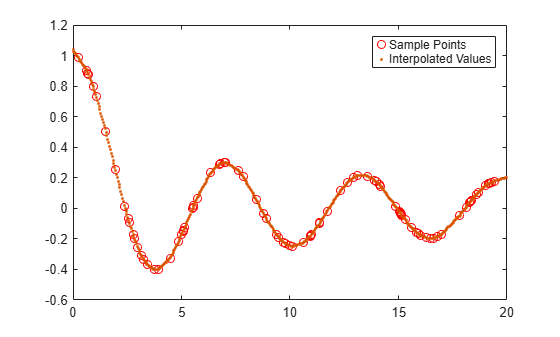Interpolate 3-D data using two methods to specify the query points.

Create and plot a 3-D data set representing the function $\mathit{z}\left(\mathit{x},\mathit{y}\right)=\frac{\mathrm{sin}\left({\mathit{x}}^{2}+{\mathit{y}}^{2}\right)}{{\mathit{x}}^{2}+{\mathit{y}}^{2}}$ evaluated at a set of gridded sample points in the range [-5,5].

[x,y] = ndgrid(-5:0.8:5);
z = sin(x.^2 + y.^2) ./ (x.^2 + y.^2);
surf(x,y,z)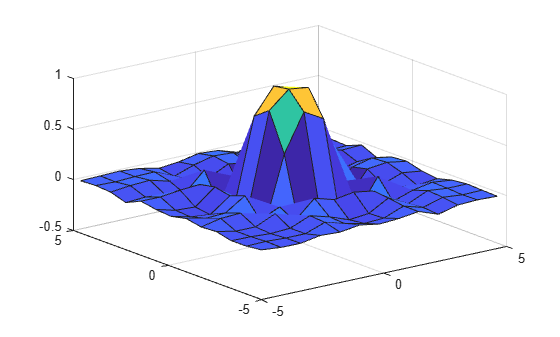Create a gridded interpolant object for the data.

F = griddedInterpolant(x,y,z);

Use a finer mesh to query the interpolant and improve the resolution.

[xq,yq] = ndgrid(-5:0.1:5);
vq = F(xq,yq);
surf(xq,yq,vq)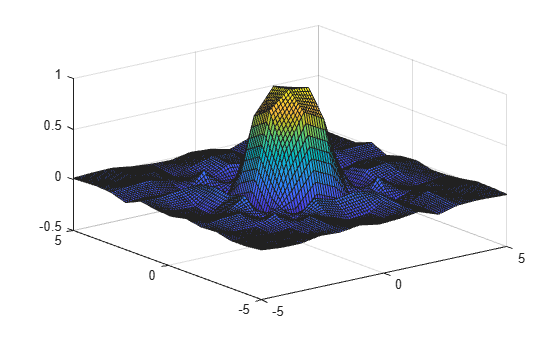In cases where there are a lot of sample points or query points, and where memory usage becomes a concern, you can use grid vectors to improve memory usage.

• When you specify grid vectors instead of using ndgrid to create the full grid, griddedInterpolant avoids forming the full query grid to carry out the calculations.

• When you pass grid vectors, they are normally grouped together as cells in a cell array, {xg1, xg2, ..., xgn}. The grid vectors are a compact way to represent the points of the full grid.Alternatively, execute the previous commands using grid vectors.

x = -5:0.8:5;
y = x';
z = sin(x.^2 + y.^2) ./ (x.^2 + y.^2);
F = griddedInterpolant({x,y},z);
xq = -5:0.1:5;
yq = xq';
vq = F({xq,yq});
surf(xq,yq,vq)Use the default grid to perform a quick interpolation on a set of sample points. The default grid uses unit-spaced points, so this interpolation is useful when the exact xy spacing between the sample points is not important.

Create a matrix of sample function values and plot them against the default grid.

x = (1:0.3:5)';
y = x';
V = cos(x) .* sin(y);
n = length(x);
surf(1:n,1:n,V)Interpolate the data using the default grid.

F = griddedInterpolant(V)
F =
griddedInterpolant with properties:

GridVectors: {[1 2 3 4 5 6 7 8 9 10 11 12 13 14]  [1 2 3 4 5 ... ]}
Values: [14x14 double]
Method: 'linear'
ExtrapolationMethod: 'linear'

Query the interpolant and plot the results.

[xq,yq] = ndgrid(1:0.2:n);
Vq = F(xq,yq);
surf(xq',yq',Vq)Interpolate coarsely sampled data using a full grid with spacing of 0.5.

Define the sample points as a full grid with range [1, 10] in both dimensions.

[X,Y] = ndgrid(1:10,1:10);

Sample $f\left(x,y\right)={x}^{2}+{y}^{2}$ at the grid points.

V = X.^2 + Y.^2;

Create the interpolant, specifying cubic interpolation.

F = griddedInterpolant(X,Y,V,'cubic');

Define a full grid of query points with 0.5 spacing and evaluate the interpolant at those points. Then plot the result.

[Xq,Yq] = ndgrid(1:0.5:10,1:0.5:10);
Vq = F(Xq,Yq);
mesh(Xq,Yq,Vq);Compare results of querying the interpolant outside the domain of F using the 'pchip' and 'nearest' extrapolation methods.

Create the interpolant, specifying 'pchip' as the interpolation method and 'nearest' as the extrapolation method.

x = [1 2 3 4 5];
v = [12 16 31 10 6];
F = griddedInterpolant(x,v,'pchip','nearest')
F =
griddedInterpolant with properties:

GridVectors: {[1 2 3 4 5]}
Values: [12 16 31 10 6]
Method: 'pchip'
ExtrapolationMethod: 'nearest'

Query the interpolant, and include points outside the domain of F.

xq = 0:0.1:6;
vq = F(xq);
figure
plot(x,v,'o',xq,vq,'-b');
legend ('v','vq')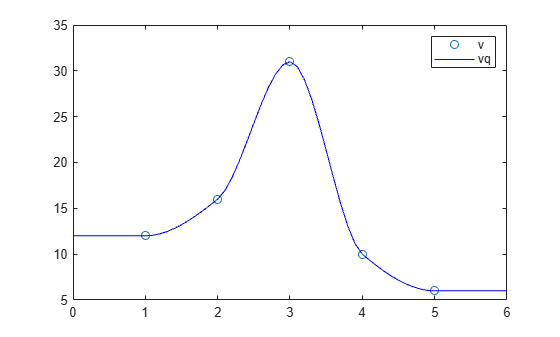Query the interpolant at the same points again, this time using the pchip extrapolation method.

F.ExtrapolationMethod = 'pchip';
figure
vq = F(xq);
plot(x,v,'o',xq,vq,'-b');
legend ('v','vq')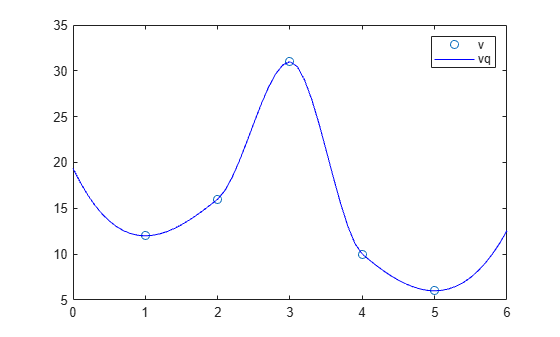Use griddedInterpolant to interpolate three different sets of values at the same query points.

Create a grid of sample points with $-5\le \mathit{X}\le 5$ and $-3\le \mathit{Y}\le 3$.

gx = -5:5;
gy = -3:3;
[X,Y] = ndgrid(gx,gy);

Evaluate three different functions at the query points, and then concatenate the values into a 3-D array. The size of V is the same as the X and Y grids in the first two dimensions, but the size of the extra dimension reflects the number of values associated with each sample point (in this case, three).

f1 = X.^2 + Y.^2;
f2 = X.^3 + Y.^3;
f3 = X.^4 + Y.^4;
V = cat(3,f1,f2,f3);

Create an interpolant using the sample points and associated values.

F = griddedInterpolant(X,Y,V);

Create a grid of query points with a finer mesh size compared to the sample points.

qx = -5:0.4:5;
qy = -3:0.4:3;
[XQ,YQ] = ndgrid(qx,qy);

Interpolate all three sets of values at the query points.

VQ = F(XQ,YQ);

Compare the original data with the interpolated results.

tiledlayout(3,2)
nexttile
surf(X,Y,f1)
title('f1')
nexttile
surf(XQ,YQ,VQ(:,:,1))
title('Interpolated f1')
nexttile
surf(X,Y,f2)
title('f2')
nexttile
surf(XQ,YQ,VQ(:,:,2))
title('Interpolated f2')
nexttile
surf(X,Y,f3)
title('f3')
nexttile
surf(XQ,YQ,VQ(:,:,3))
title('Interpolated f3')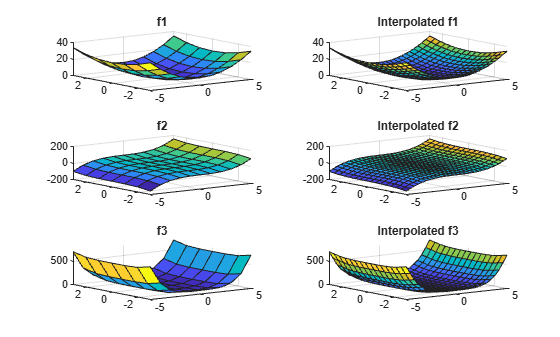expand all

Tips

• It is quicker to evaluate a griddedInterpolant object F at many different sets of query points than it is to compute the interpolations separately using interp1, interp2, interp3, or interpn. For example:

% Fast to create interpolant F and evaluate multiple times
F = griddedInterpolant(X1,X2,V)
v1 = F(Xq1)
v2 = F(Xq2)

% Slower to compute interpolations separately using interp2
v1 = interp2(X1,X2,V,Xq1)
v2 = interp2(X1,X2,V,Xq2)